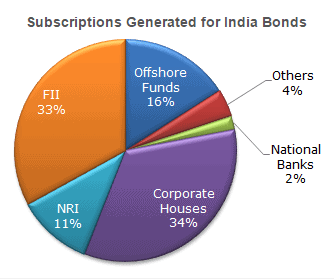Courses

# Test: Pie Chart- 4

## 5 Questions MCQ Test General Aptitude for GATE 2020 | Test: Pie Chart- 4

Description
This mock test of Test: Pie Chart- 4 for LR helps you for every LR entrance exam. This contains 5 Multiple Choice Questions for LR Test: Pie Chart- 4 (mcq) to study with solutions a complete question bank. The solved questions answers in this Test: Pie Chart- 4 quiz give you a good mix of easy questions and tough questions. LR students definitely take this Test: Pie Chart- 4 exercise for a better result in the exam. You can find other Test: Pie Chart- 4 extra questions, long questions & short questions for LR on EduRev as well by searching above.
QUESTION: 1

### The following pie chart shows the amount of subscriptions generated for India Bonds from different categories of investors.In the corporate sector, approximately how many degrees should be there in the central angle ?

Solution:

34 x 3.6 = 122.4 (since 1% = 3.6 degrees)

QUESTION: 2

### The following pie chart shows the amount of subscriptions generated for India Bonds from different categories of investorsIf the investment by NRI's are Rs 4,000 crore, then the investments by corporate houses and FII's together is:

Solution:

(67/11) x 4000 = 24 363.6364

QUESTION: 3

### The following pie chart shows the amount of subscriptions generated for India Bonds from different categories of investors.What percentage of the total investment is coming from FII's and NRI's ?

Solution:

(33 + 11) = 44

QUESTION: 4

The following pie chart shows the amount of subscriptions generated for India Bonds from different categories of investors.If the total investment other than by FII and corporate houses is Rs 335,000 crore, then the investment by NRI's and Offshore funds will be (approximately) ?

Solution:

Investment other than NRI and corporate houses is 33% = 335000. Also, investment by offshore funds and NRI's is equal to 27%.

Hence, 27 x 335,000/33 = 274 090.909

QUESTION: 5

The following pie chart shows the amount of subscriptions generated for India Bonds from different categories of investors.If the total investment flows from FII's were to be doubled in the next year and the investment flows from all other sources had remained constant at their existing levels for this year, then what would be the proportion of FII investment in the total investment into India Bonds next year (in US \$ millions) ?

Solution:

FII's currently account for 33 out of 100.

If their value is doubled and all other investments are kept constant then their new value would be 66 out of 133 = approximately equal to 50%